WHAT IS an amoeba?

Oleg Viro                 Notices of the AMS, 49, No. 8, September 2002, 916-917.

In mathematical terminology the word amoeba is a recent addition.1 It was introduced by I.M.Gelfand, M.M.Kapranov and A.V.Zelevinsky in their book  in 1994. A mathematical amoeba falls short in being similar to its biological prototype. In the simplest case, it is a region in
, which may pretend to be a picture of amoeba: a body with several holes (vacuoles) and straight narrowing tentacles (pseudopods) reaching to infinity.

A planar amoeba is the image of the zero locus of a polynomial in two variables under the map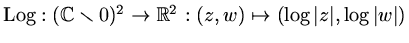For example, here are the amoebas of a linear polynomial and of a quartic polynomial.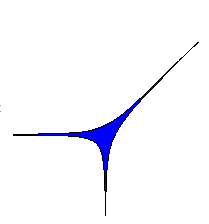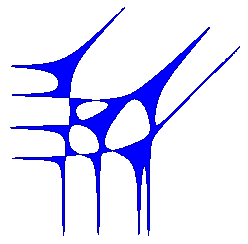The zero locus of a polynomial in two variables is called a plane complex algebraic curve. This is a surface in the 4-space
defined by an equation
, where
is a polynomial
with complex coefficients
. The minimal convex polygon
which contains all points
corresponding to non-zero coefficients of the equation is called the Newton polygon of. It represents the geometry of the equation, and, as we will see, its geometry is closely related to geometry of the corresponding complex curve
and its amoeba
.

An amoeba reaches infinity by several tentacles. Each tentacle accommodates a ray, and narrows exponentially fast towards it. Thus there is only one ray in a tentacle. The ray is orthogonal to a side of the Newton polygon and directed along an outward normal of the side. For each side ofthere is at least one tentacle associated to it. The maximal number of such tentacles is a sort of lattice length of the side: the number of pieces to which the side is divided by integer lattice points (i.e., points with integer coordinates). We observe this in the two amoebas above, whoe respective Newton polygons we display below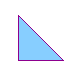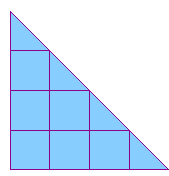Each connected component of amoeba's complement
is convex. Besides components lying between tentacles, there can be bounded components. The number of bounded components is at most the number of interior integer lattice points of, and hence the total number of components ofis at most the number of all integer lattice points of. Each component corresponds to some integer lattice point of. To establish this correspondence, take a point in a component of, and consider its preimage under the map
. The preimage is a torus, it consists of points whose complex coordinates have fixed absolute values, but varying arguments. On the torus there are circles: meridians and parallels, consisting of points with one of the coordinates, fixed. Consider a curve
, on which the abscissa
is fixed. Inthere is a disk
bounded by. Let us count the intersections ofwith the complex curve
counting intersection points with multiplicities (so this is rather homological intersection number
, or, if you like, the linking number
). Denote the intersection number by
and the number which is defined similarly, but starting with a curve on the torus where the ordinate
is fixed, by
. These numbers do not depend on choices made after choosing a connected component of: varying the curves on the torus and even the torus itself do not affect them. The pointbelongs to. This is the point corresponding to the component ofwe started with. Different components ofgive rise to different integer lattice points of. It may happen that some integer lattice points ofdo not correspond to any component. Only vertices ofcorrespond to components necessarily. Any collection of integer lattice points of, which includes all vertices, is realizable by the amoeba of an appropriate algebraic curve with this Newton polygon.

Although a planar amoeba is not bounded, its area is finite. Moreover,
Complex curves whose amoebas have the extremal area are very special. In particular,has the maximal number of components. Multiplication of the standard coordinates inby appropriate complex numbers makes such a curve real, i.e. defined by a polynomial equations with real coefficients. The geometry of the real part of this curve is also very special. It has the maximal number of components among curves with given Newton polygon and the components are positioned with respect to each other in a special simple way.

These real curves are remarkable from many viewpoints. They were discovered by A. Harnack in 1876 when he constructed real algebraic plane projective curves with the maximal number of components for each degree. Only one component of a Harnack curve meets the coordinate axes (including the line of infinity), and the intersections with the axes lie on disjoint arcs of this component. Consideration of amoebas allowed G.Mikhalkin to prove that any real curve with these properties must be topologically isotopic to a Harnack curve.

One of the main analytic tools used in study of amoebas is a remarkable Ronkin function
. For a polynomial, it is defined by
Ifis a monomial
, thenis a linear function,
with gradient
. For a general, the Ronkin function is convex. On each component ofit behaves like the Ronkin function of a monomial: it is linear, and its gradient is the corresponding integer point of. The maximum of these linear functions is a piecewise linear convex function. The set where it is not differentiable is a union of segments and rays contained in the amoeba and being its deformation retract. It is called the spine of
.

Logarithmic coordinates and amoebas disclose a piecewise linear stream in the nature of algebraic geometry. There is a non-archimedian version of amoebas which brings these ideas to algebraic varieties over other fields. There is also a similar theory in higher dimensions. The notion of an algebraic curve is replaced by the notion of an algebraic variety, and the Newton polygon becomes a Newton polytope. Amoebas provide a new way to visualize complex algebraic varieties. Looking at an amoeba, one can see handles of complex curves and cycles in high dimensional varieties, watch degenerations and build more complicated varieties from simple ones.

The theory of amoebas is a fresh and beautiful field of research, still quite accessible to a newcomer, where exciting discoveries are still ahead. The impressive results described above were obtained during a short period of about 8 years by few people. The first remarks belong to I.M.Gelfand, M.M.Kapranov and A.V.Zelevinsky. Relations between components ofand integer lattice points ofhave been discovered by M. Forsberg, M. Passare and A. Tsikh. The Spine of amoeba, Ronkin function, estimate of area are due to H.Rullgård and M.Passare. Homological interpretations and relations to real algebraic geometry are due to G. Mikhalkin. I enjoyed the feast. About 20 years ago I found a way to construct real algebraic curves by a sort of gluing curves to each other. I heard that this gluing and use of logarithmic coordinates in its description, being replanted to the complex soil, have motivated introduction of amoebas. A version of the gluing is used to glue amoebas.

Bibliography

1
Mikael Forsberg, Mikael Passare, August Tsikh, Laurent determinants and arrangements of hyperplane amoebas, Advances in Math. 151 (2000), 45-70.
2
I. M. Gelfand, M. M. Kapranov, A. V. Zelevinsky, Discriminants, resultants, and multidimensional determinants. Mathematics: Theory & Applications. Birkhäuser Boston, Inc., Boston, MA, 1994. x+523 pp.
3
Grigory Mikhalkin, Real algebraic curves, moment map and amoebas, Ann. of Math. 151 (2000), no. 1, 309-326.

1 I was told that in mathematical logic amoebas were known for more than twenty years. However, they belong to an entirely different kind of mathematical microbes, and have never bitten me, so I cannot tell you about them.